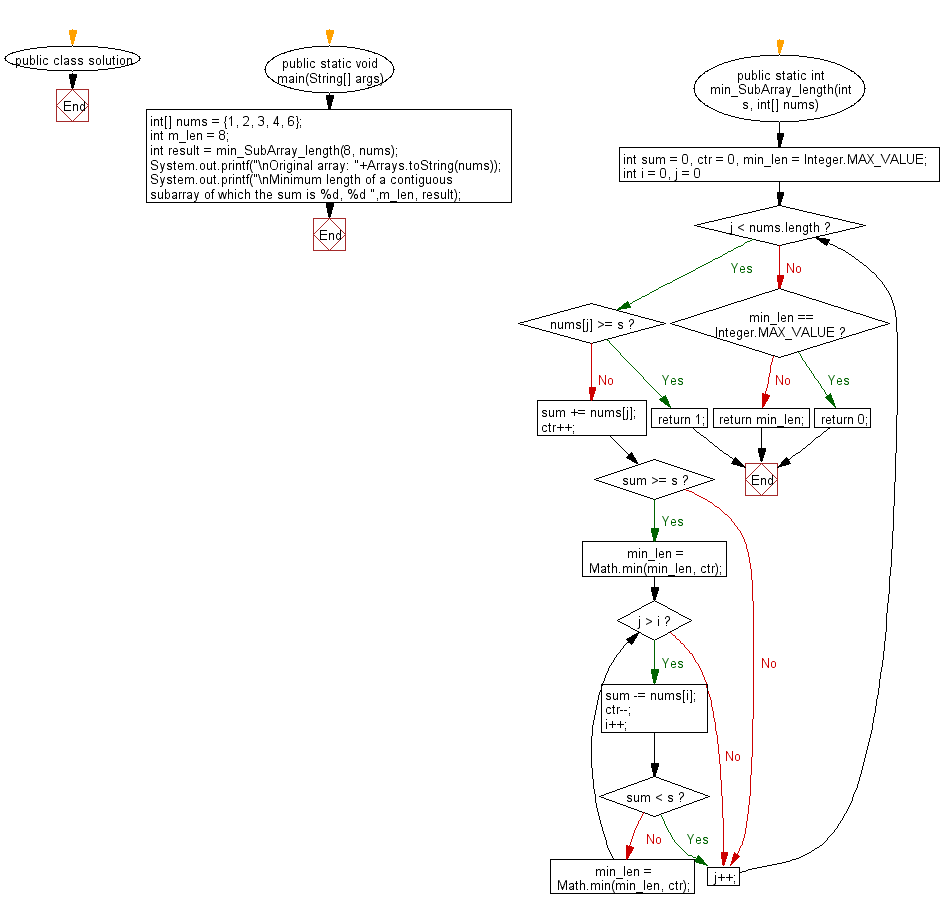﻿ Java: Shortest length of a subarray according to the sum# Java Array Exercises: Find the smallest length of a contiguous subarray of which the sum is greater than or equal to specified value

## Java Array: Exercise-70 with Solution

Write a Java program to find the smallest length of a contiguous subarray of which the sum is greater than or equal to specified value. Return 0 instead.

Example:
Input :
nums = {1, 2, 3, 4, 6}
Output:
Minimum length of a contiguous subarray of which the sum is 8, 2

Sample Solution:

Java Code:

``````import java.util.Arrays;
public class solution {
public static void main(String[] args)
{
int[] nums = {1, 2, 3, 4, 6};
int m_len = 8;
int result = min_SubArray_length(8, nums);
System.out.printf("\nOriginal array: "+Arrays.toString(nums));
System.out.printf("\nMinimum length of a contiguous subarray of which the sum is %d, %d ",m_len, result);
}

public static int min_SubArray_length(int s, int[] nums) {
int sum = 0, ctr = 0, min_len = Integer.MAX_VALUE;
for (int i = 0, j = 0; j < nums.length; ) {
if (nums[j] >= s) {
return 1;
} else {
sum += nums[j];
ctr++;
if (sum >= s) {
min_len = Math.min(min_len, ctr);
while (j > i) {
sum -= nums[i];
ctr--;
i++;
if (sum < s) break;
min_len = Math.min(min_len, ctr);
}
}
}
j++;
}
if (min_len == Integer.MAX_VALUE) {
return 0;
}
return min_len;
}
}
```
```

Sample Output:

```Original array: [1, 2, 3, 4, 6]
Minimum length of a contiguous subarray of which the sum is 8, 2
```

Flowchart:Java Code Editor:

Improve this sample solution and post your code through Disqus

What is the difficulty level of this exercise?

Test your Programming skills with w3resource's quiz.

﻿

## Java: Tips of the Day

getEnumMap

Converts to enum to Map where key is the name and value is Enum itself.

```public static <E extends Enum<E>> Map<String, E> getEnumMap(final Class<E> enumClass) {
return Arrays.stream(enumClass.getEnumConstants())
.collect(Collectors.toMap(Enum::name, Function.identity()));
}
```

Ref: https://bit.ly/3xXcFZt